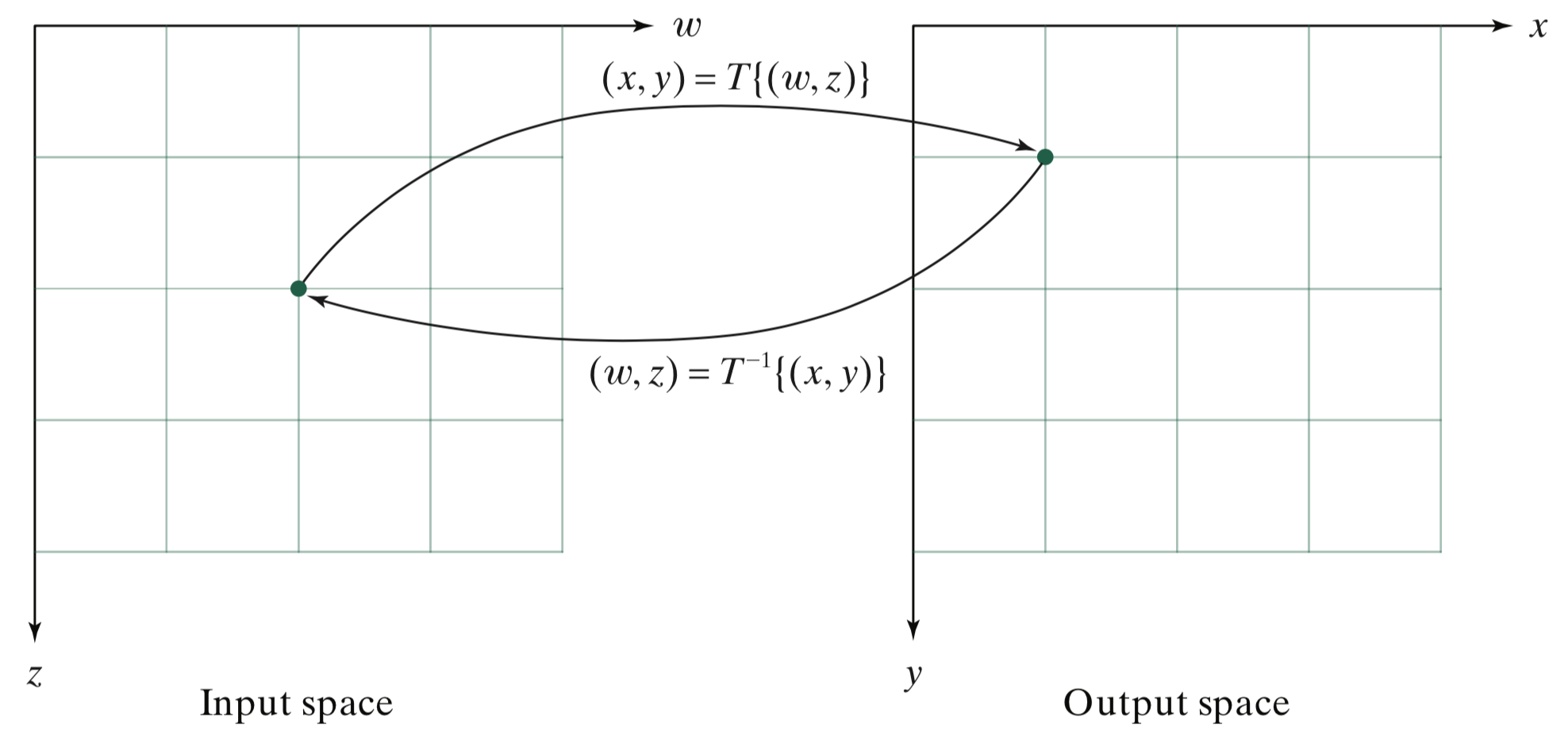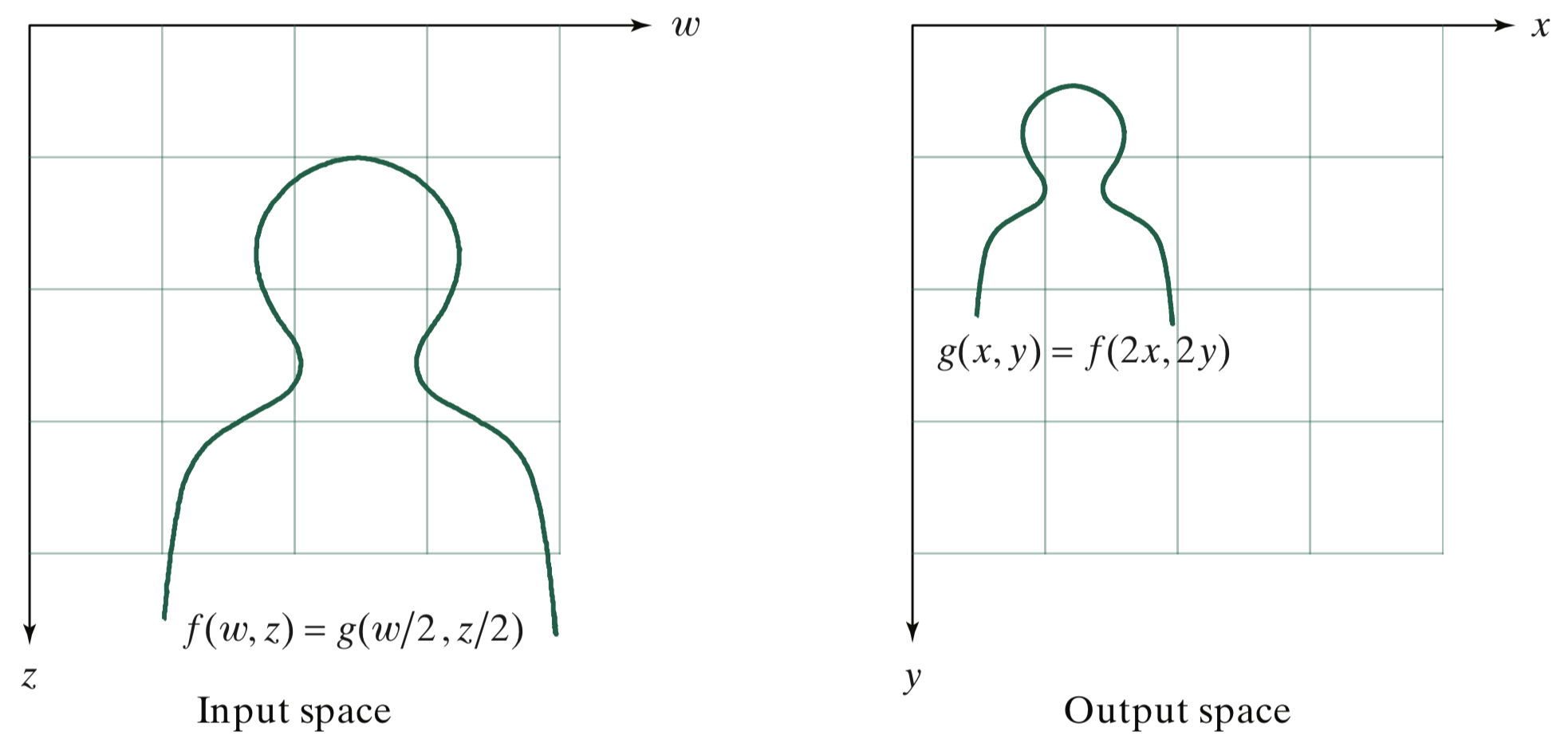# Geometric Transformation of Points – Getting Started

I like to think of geometric transformations of images (stretching, shrinking, rotating, etc.) by starting with the geometric transformations of points. You could think of a geometric point transformation as just moving a point from one location to another, like this:But that terminology quickly gets a little confusing. (What does it mean to move a point in a coordinate system to another location?) It helps to get little more systematic in our notation and thinking. Let's try defining a geometric transformation of a point as a mapping from a location in one coordinate system to a location (usually different) in another coordinate system. Here's a diagram to illustrate:DIPUM3E Figure 6.1

The Cartesian coordinate system $(w,z)$ is called the input space, and the Cartesian coordinate system $(x,y)$ is called the output space. (Note that Image Processing Toolbox documentation uses $(u,v)$ for the input space.) The mapping $T\{\cdot\}$ is called the forward mapping, or forward transformation. The mapping $T^{-1}\{\cdot\}$ is called the inverse mapping, or inverse transformation. In the diagram above, $(x,y) = T\{(w,z)\} = (w/2,z/2)$.

To give you a hint of where I'm going with this, imagine transforming a bunch of points according to the mapping $(x,y) = T\{(w,z)\} = (w/2,z/2)$. Specifically, imagine transforming all the points along the curve on the left, below:DIPUM3E Figure 6.2

On the right, you can see where the mapping moves all those points. And, if you squint and use your imagine, you can start to see how this idea might form the basis for shrinking an image.

More on this later.

Published with MATLAB® R2020a

|

### コメント

コメントを残すには、ここ をクリックして MathWorks アカウントにサインインするか新しい MathWorks アカウントを作成します。